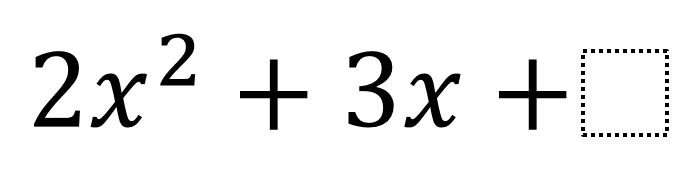Home > High School: Algebra > Factoring Quadratics With Undefined C

# Factoring Quadratics With Undefined C

Directions: Fill in the blanks by finding the largest and smallest integers that will make the quadratic expression factorable.### Hint

How would you represent this problem using visually (such as by using algebra tiles)?
What patterns are you observing for the maximum and minimum values of C?

### Answer

The largest C can be is 1. You can continue to find smaller and smaller values of C, so the smallest value of C is negative infinity.

Source: Robert Kaplinsky

## Writing Equivalent Polynomial Expressions

Directions: Use the digits 1-9, at most one time each, to create a true statement. …

### One comment

1.if you are OK with fractions..i.e. factoring does not require integers the value of c = 1 1/8 also works.

Also if you factor with complex numbers there are infinite values of c > 1.

c = 2 yields solutions of x = {-3+/- Sqrt (-7) } 4 , which in turn can be factors…ugly ones, but factors none the less.